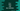# fabs and abs methods in C++ explanation with example## fabs and abs methods in C++:

Both fabs and abs methods are defined in the cmath header file. Let me show you the definitions for both to give you a clear understanding.

### fabs() method:

fabs method is defined as like below:

``````double fabs (double x);
float fabs (float x);
long double fabs (long double x);
double fabs (T x);``````

This method returns the absolute value of a number. In C++ 11, new overloads are added. T is used for integral type. It casts that value to a double.

#### Return value:

It returns the absolute value for the provided number.

### Example of fabs:

Let’s take an example of fabs:

``````#include <iostream>
#include <cmath>

int main ()
{

std::cout << "fabs (3.45) = " << std::fabs (3.45) << '\n';
std::cout << "fabs (-5.5)  = " << std::fabs (-5.5) << '\n';
std::cout << "fabs (-5.59)  = " << std::fabs (-5.59) << '\n';
return 0;
}``````

It will print:

``````fabs (3.45) = 3.45
fabs (-5.5)  = 5.5
fabs (-5.59)  = 5.59``````

### abs() method:

abs method is defined as like below:

``````double abs (double x);
float abs (float x);
long double abs (long double x);
double abs (T x);``````

It is similar to fabs and it returns the absolute value for a given number. Starting from C++ 11, additional overloads are added to the cmath header for integral types. The integral types are casted to a double.

#### Return value:

It returns the absolute value for a given number.

In C, abs is defined in stdlib.h header file and it works only on integer values.

### Example:

The below example uses abs:

``````#include <iostream>
#include <cmath>

int main ()
{

std::cout << "abs (3.45) = " << std::abs (3.45) << '\n';
std::cout << "abs (-5.5)  = " << std::abs (-5.5) << '\n';
std::cout << "abs (-5.59)  = " << std::abs (-5.59) << '\n';
return 0;
}``````

It will print:

``````abs (3.45) = 3.45
abs (-5.5)  = 5.5
abs (-5.59)  = 5.59``````